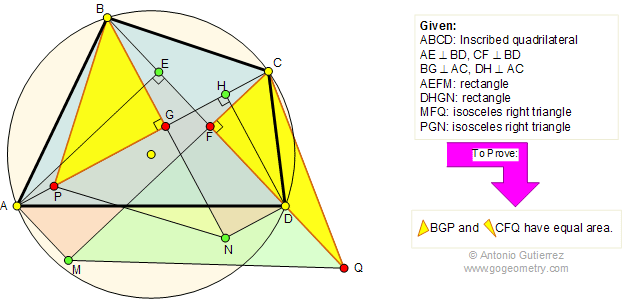Problem 293: Inscribed Quadrilateral, Perpendicular, Rectangle, Isosceles Right triangle, Area, Similarity. Level: High School, College, SAT Prep.

The figure shows a quadrilateral ABCD inscribed in a circle. AE and CF are perpendicular to BD, and BG and DH are perpendicular to AC. AEFM and DHGN are rectangles. MFQ and PGN are isosceles right triangles. Prove that triangles BGP and CFQ have equal area.Geometry problem solving is one of the most challenging skills for students to learn. When a problem requires auxiliary construction, the difficulty of the problem increases drastically, perhaps because deciding which construction to make is an ill-structured problem. By “construction,” we mean adding geometric figures (points, lines, planes) to a problem figure that wasn’t mentioned as "given."